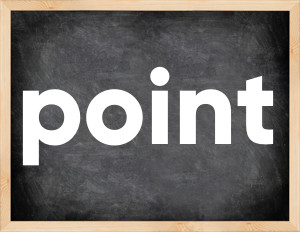# Point past tenseThe English verb 'point' is pronounced as [pɔɪnt].
Related to: regular verbs.
3 forms of verb point: Infinitive (point), Past Simple - (pointed), Past Participle - (pointed).

## Here are the past tense forms of the verb point

👉 Forms of verb point in future and past simple and past participle.
❓ What is the past tense of point.

## Point: Past, Present, and Participle Forms

Base Form Past Simple Past Participle
point [pɔɪnt]

pointed [ˈpɔɪntɪd]

pointed [ˈpɔɪntɪd]

## What are the 2nd and 3rd forms of the verb point?

🎓 What are the past simple, future simple, present perfect, past perfect, and future perfect forms of the base form (infinitive) 'point'?

### Learn the three forms of the English verb 'point'

• the first form (V1) is 'point' used in present simple and future simple tenses.
• the second form (V2) is 'pointed' used in past simple tense.
• the third form (V3) is 'pointed' used in present perfect and past perfect tenses.

## What are the past tense and past participle of point?

The past tense and past participle of point are: point in past simple is pointed, and past participle is pointed.

### What is the past tense of point?

The past tense of the verb "point" is "pointed", and the past participle is "pointed".

### Verb Tenses

Past simple — point in past simple pointed (V2).
Future simple — point in future simple is point (will + V1).
Present Perfect — point in present perfect tense is pointed (have/has + V3).
Past Perfect — point in past perfect tense is pointed (had + V3).

### point regular or irregular verb?

👉 Is 'point' a regular or irregular verb? The verb 'point' is regular verb.

## Examples of Verb point in Sentences

•   She wants you to point the target (Present Simple)
•   The statue pointed to the secret route (Past Simple)
•   The Secretary-General has pointed to underlying conditions (Present Perfect)
•   She pointed to a chair for me. (Past Simple)
•   The boss pointed to the door to her. (Past Simple)
•   He stood up and suddenly pointed his finger at me. (Past Simple)
•   He will point the method of treatment. (Future Simple)
•   He pointed me to an empty parking space near the supermarket. (Past Simple)
•   She took the camera and pointed it at me. (Past Simple)
•   He pointed out all the flaws in my plan. (Past Simple)

Along with point, words are popular view and sport.

Verbs by letter: , , , , , , , , , , , , , , , , , , , , , , , , .